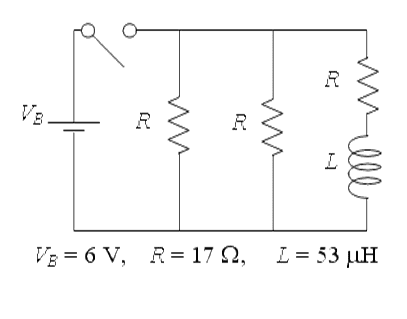# RL circuit with three resistors

• syhpui2

## Homework Statement

http://i.imgur.com/22jq3.pngThree identical resistors are connected to a battery, an inductor, and a switch as shown in the figure. (The values of all circuit elements are given below the figure.) The switch has been open for a very long time, and then it is closed at time t = 0.

Part A

Immediately after the switch is closed, the voltage VL across the inductor is
(a) VL = 0 V
(b) VL = 6 V Correct
(c) Neither of the above answers is correct.

Part B

After the switch has been closed for a very long time, how much energy EL is stored in the inductor?
(a) EL = 3.30 × 10-6 J Correct
(b) EL = 5.62 × 10-6 J
(c) EL = 8.63 × 10-6 J
(d) EL = 1.07 × 10-5 J
(e) EL = 1.12 × 10-5 J

Part C
After the switch has been closed for a very long time, a steady current has been established through the inductor. The switch is then reopened. What fraction of this current, fI, remains after a time interval of Δt = 3 μsec has elapsed?
(a) fI = 0.0521
(b) fI = 0.0926
(c) fI = 0.158
(d) fI = 0.201
(e) fI = 0.236 Correct

KVL, KCL

## The Attempt at a Solution

Part A,
can i just say regardless of any situation, VL is equal to Vbattery immdiately after switch is closed?

Part B,
I can't find the current across L. in this case.

Part C,

Thx

You need the relation between voltage and current across the inductor.

ehild

A: When you first try to push current through an inductor, does it act like a short circuit, an open circuit, or a resistive circuit?

"current across L" is an odd statement, since current is never "across" anything. Current flows THROUGH things due to voltage ACROSS things. This is NOT semantics, it is integral to how electricity works.

A: When you first try to push current through an inductor, does it act like a short circuit, an open circuit, or a resistive circuit?

"current across L" is an odd statement, since current is never "across" anything. Current flows THROUGH things due to voltage ACROSS things. This is NOT semantics, it is integral to how electricity works.

No current? I think...